### Home > A2C > Chapter 10 > Lesson 10.2.6 > Problem10-149

10-149.
1. Rewrite each equation in graphing form and identify the key points. Homework Help ✎

1.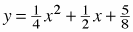2.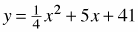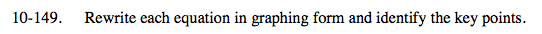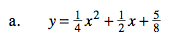Multiply by 4.

$4\textit{y}=\textit{x}^{2}+2\textit{x} + \frac{5}{2}$

$\text{Now complete the square and multiply by \frac{1}{4}.}$

$\textit{y}=\frac{1}{4}(\textit{x}^{2}+2\textit{x}+1)+\frac{3}{8}$

$\textit{y}=\frac{1}{4}(\textit{x}+1)^{2}+\frac{3}{8}$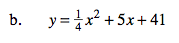See part (a).